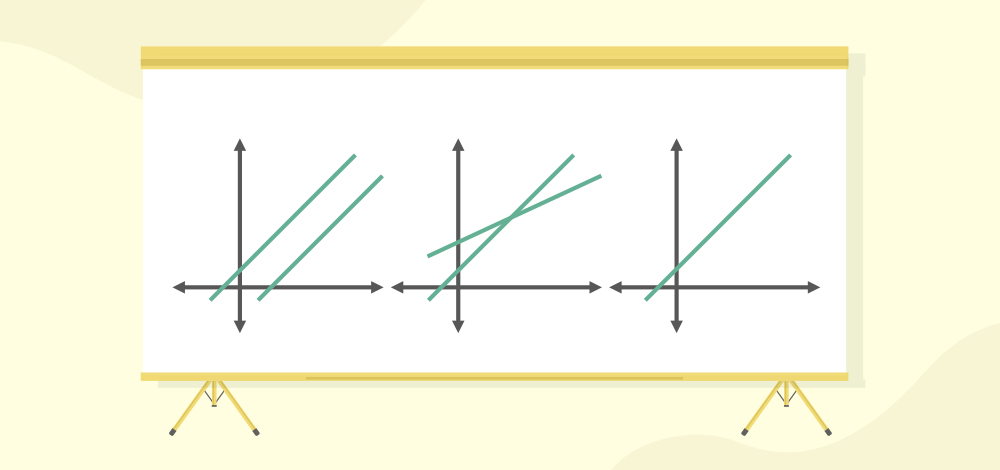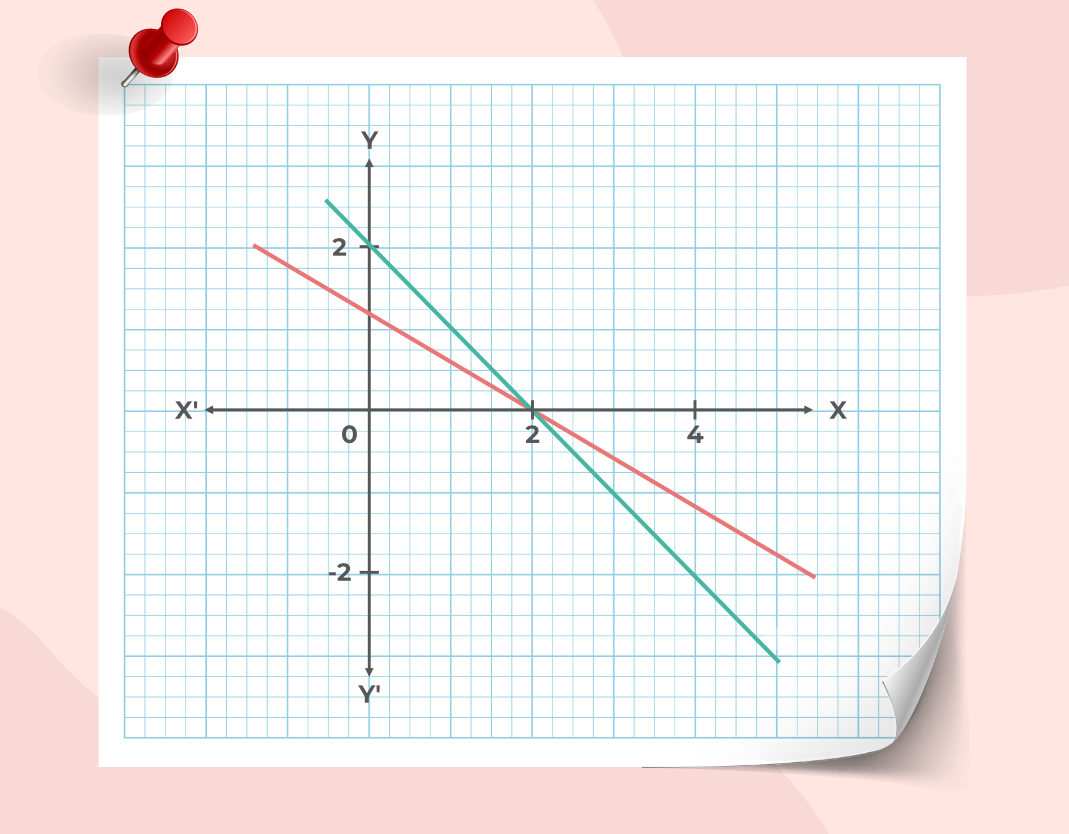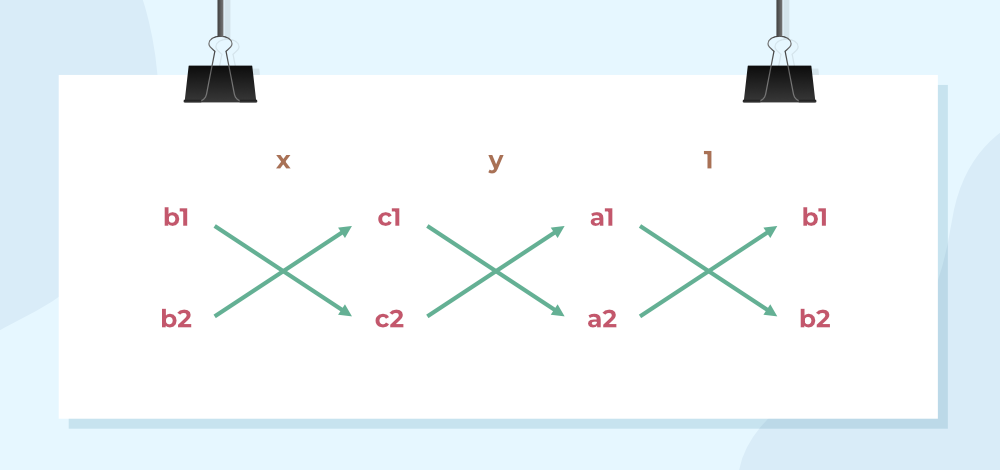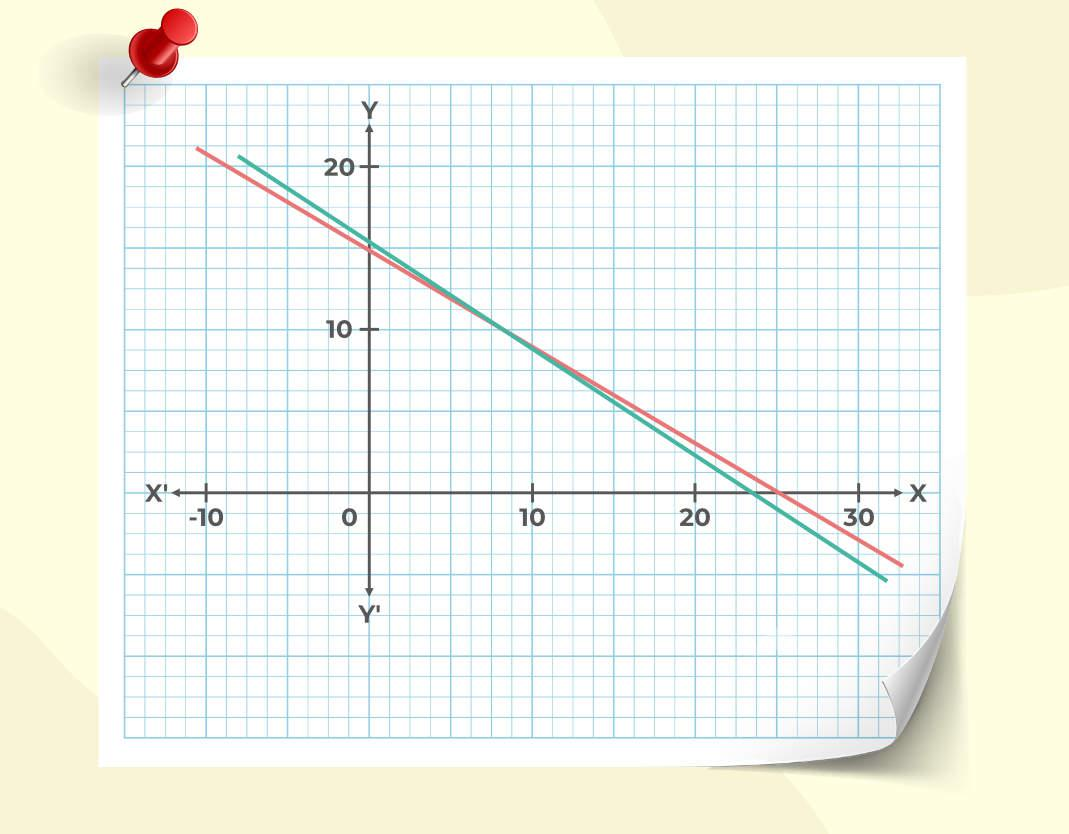GeeksforGeeks App
Open AppBrowser
Continue

# Pair of Linear Equations in Two Variables

Linear Equation in two variables are equations with only two variables and the exponent of the variable is 1. This system of equations can have a unique solution, no solution, or an infinite solution according to the given initial condition. Linear equations are used to describe a relationship between two variables. Sometimes in some situations, we don’t know the values of the variables we want to observe. So, then we formulate the equations describing how they behave and solve them. The Number of equations obtained should be equal to the number of variables.

Let’s learn about the Pair Of Linear Equation In Two Variables and their solution in this article.

## Pair Of Linear Equation In Two Variables

A linear equation is defined as,

ax + by + c = 0

where
a, b and c are real numbers
a and b both are not zero

A pair is formed by two such linear equations. It can be represented as,

a1x + b1y + c1 = 0

a2x + b2y + c2 = 0

where,
a1, b1, c1, a2, b2 and c2 are real numbers.

Since a linear equation represents a line on the Cartesian plane. A pair represents two lines on the Cartesian plane. The solution to this system will be the points that satisfy both of these equations. There are three possibilities in such a system,

### No Solution

If lines are parallel to each other then they have no solution. For example, 2x + 3y = 11 and 2x + 3y = 13 are parallel lines and they have no solution.

### Unique Solution

If two lines intersect each other at any point then they have a unique solution. For example, 2x + 3y = 11 and 3x + 2y = 11 are intersecting lines and they have a unique solution.

### Infinitely Many Solutions

If two lines are overlapping with each other then they have infinite solutions. For example, 2x + 3y = 11 and 4x + 6y = 22 are overlapping lines and they have infinite solutions.## Representation of Pair Of Linear Equation In Two Variables

The pair of linear equations can be solved and represented by two methods which include,

• Graphical Method
• Algebraic Method

The general form of a pair of linear equations in two variables is,

a1x + b1y + c1 = 0

a2x + b2y + c2 = 0

where,
a1, b1, c1, a2, b2 and c2 are real numbers
a12 + b12 ≠ 0
a22+ b22 ≠ 0

The pair of linear equations have three conditions,

• If a1/a2 ≠ b1/b2 the pair of linear equations is consistent.
• If a1/a2 = b1/b2 ≠ c1/c2 the pair of linear equations is inconsistent.
• If a1/a2 = b1/b2 = c1/c2 the pair of linear equations is dependent and consistent.

If we compare the coefficients a1, b1, c1, a2, b2 and c2  of different pairs of equations which have a unique solution, infinite solutions, and no solutions. We obtain the results given below in the table.

For two lines,

a1x + b1y + c1 = 0

a2x + b2y + c2 = 0

## Graphical Representation

In this method, we represent the equations on the graph and find out their intersection through it. We look for the points which are common to both lines, sometimes there is only a point like that, but it might also happen that there are no solutions or infinite solutions.

Example: Find out the intersection of the following lines.

3x + 5y = 6

x + y = 2

Solution:

We will plot both the lines on the graph.These lines intersect at (2,0).

For more, visit: Graph of Linear Equations in Two Variables

## Algebraic Methods of Solving a Pair of Linear Equations

Various methods of Solving a Pair of Linear Equations are,

• Substitution Method
• Elimination Method
• Cross-Multiplication Method

### Substitution Method

In this method, we use one equation to express a variable in terms of the other variable thereby reducing the number of variables in the equation. Then we substitute that expression into the other equation that is given to us.

Example: Solve the following pair of equations with the substitution method.

x + y = 3

3x + y = 16

Solution:

Let’s pick the equation

x + y = 3

x = 3 – y

Substituting the value of x in the other equation,

3x + y = 16

3(3 – y) + y = 16

9 – 3y + y = 16

-2y =7

y = -7/2

### Elimination Method

This method is sometimes more convenient than the substitution method. In this method, we eliminate one variable by multiplying and adding equations with suitable constants, this is done to eliminate one variable, and when the equation is left with just one variable, it can easily be solved.

Example: Solve the following equations with the elimination method.

x + y = 3

x – y = 5

Solution:

We have two equations,

x + y = 3  …….(1)

x – y = 5 …… (2)

Adding the equation (1) and (2) to eliminate the variable -y.

2x = 8

x = 4

Substituting the value of x in equation (1)

4 + y = 3

y = -1

### Cross-Multiplication Method

This method looks more complex than the other methods, but it is one of the most efficient ways to solve linear equations. Let’s say the two lines whose equation is,

a1x + b1y + c1 = 0

a2x + b2y + c2 = 0

In this cross-multiplication method,The solution is given by,Example: Solve the following equations with the elimination method.

2x + 3y = 46

3x + 5y = 74

Solution:

a1 = 2, a2 = 3, b1 = 3, b2 = 5, c1 = 46 and c2 = 74x = 8 and y = 10

## Solved Examples on Linear Equations in Two Variables

Example 1: Solve the following pair of equations graphically:

2x + 3y = 46

3x + 5y = 74

Solution:

We need to plot them of graph separately and then look at their intersection.This graph intersects are (10,8)

Example 2: Solve the following pair of linear equations with the substitution method.

5x + 4y = 20

x + 2y = 4

Solution:

We have to solve these two equations

5x + 4y = 20

x + 2y = 4

Let’s we pick the second equation,

x = 4 – 2y

Now substituting the value of x in the other equation.

5(4 – 2y) + 4y = 20

20 – 10y + 4y = 20

-6y = 0

y = 0

Finding out the value of x by substituting the value of y in the equation,

x = 4 – 2y

x = 4

(4, 0) is the solution to this pair of linear equations.

Example 3: Solve the following equations with the elimination method.

4x + 5y = 20

8x + 2y = 5

Solution:

Let the equations be,

4x + 5y = 20 ……..(1)

8x + 2y = 5 ….. (2)

We need to eliminate one of the variables here from these two equations,

Multiply equation (1) with 2 and subtract it with (2).

2 x(1) -(2)

8x + 10y = 40 ….. 2 x(1)

8x + 2y = 5 …..(2)

Subtracting both of these,

8y = 35

y =Substituting this value in equation (1)## FAQs on Linear Equations in Two Variables

### Question 1: What is Linear Equation in Two Variables?

A linear equation is an equation with degree 1. A linear equation in two variables is a type of linear equation in which there are 2 variables present. For example, 4x – 3y = 5, 3a – b = 4 etc.

### Question 2: How to solve Linear Equation in Two Variables?

Linear Equations in Two Variables are solved by two methods,

• Graphical Method
• Algebraic Method

### Question 3: What are examples of Linear Equations in Two Variables?

The standard form for linear equations in two variables is ax + by = c. For example, 4x + 7y = 5

### Question 4: How many solutions are there for linear equations in two variables?

A linear equation in two variables can have a unique solution, no solution and an infinitely solution.